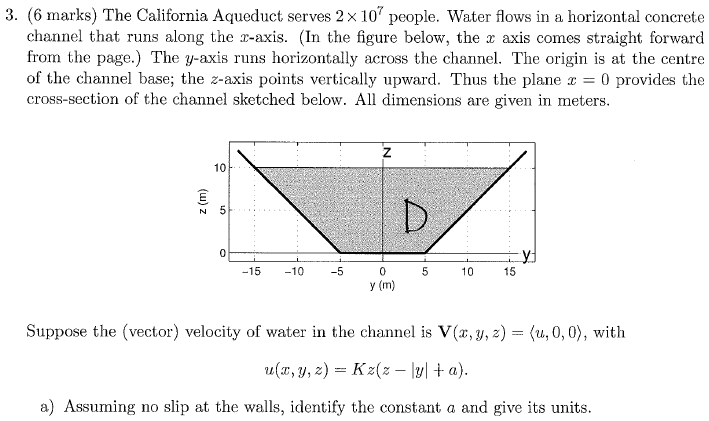# Finding the constant in the velocity function?

## Homework Statement

I am confused about how to get the constant a in this equation.## The Attempt at a Solution

Apparently you can get the constant a from the wall equations. However I don't see how the wall equations are related to the velocity function. The velocity function could have been ANY arbitrary function right? So how can it be related to something else?

SammyS
Staff Emeritus
Homework Helper
Gold Member

## Homework Statement

I am confused about how to get the constant a in this equation.## The Attempt at a Solution

Apparently you can get the constant a from the wall equations. However I don't see how the wall equations are related to the velocity function. The velocity function could have been ANY arbitrary function right? So how can it be related to something else?
What does "no slip at the walls" mean?

Does it mean that the water flow right along each wall is zero?

Ray Vickson
Homework Helper
Dearly Missed

## Homework Statement

I am confused about how to get the constant a in this equation.## The Attempt at a Solution

Apparently you can get the constant a from the wall equations. However I don't see how the wall equations are related to the velocity function. The velocity function could have been ANY arbitrary function right? So how can it be related to something else?

No slip at the walls means that u = 0 when (y,z) lies on either wall. We also have u = 0 when y = a + |z|.

No slip at the walls means that u = 0 when (y,z) lies on either wall. We also have u = 0 when y = a + |z|.

Wow cool, thank you :)